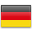# Azure Databricks| Working with Unit Tests

## Introduction

### Problem

Like any other program, Azure Databricks notebooks should be tested automatically to ensure code quality.

Using standard Python Test Tools is not easy because these tools are based on Python files in a file system. And a notebook doesn’t correspond to a Python file.

### Solution

To enable automated testing with unittest (documentation), we proceed as follows:

• Create a test class that contains all the tests you want
• Execution of all defined tests

## Create Notebook with the Code

We will create a simple Notebook for our test.

This notebook will implement a simple calculator, so that we can test the basic calculator operations like add ad multiply.

Create a new Notebook with the name Calculator:

```class Calculator:

def __init__(self, x = 10, y = 8):
self.x = x
self.y = y

def add(self, x = None, y = None):
if x == None: x = self.x
if y == None: y = self.y

return x+y

def subtract(self, x = None, y = None):
if x == None: x = self.x
if y == None: y = self.y

return x-y

def multiply(self, x = None, y = None):
if x == None: x = self.x
if y == None: y = self.y

return x*y

def divide(self, x = None, y = None):
if x == None: x = self.x
if y == None: y = self.y

if y == 0:
raise ValueError('cannot divide by zero')
else:
return x/y```

The notebook should look like this

To use this class, write the following lines:

```c = Calculator()
print(c.add(20, 10), c.subtract(20, 10), c.multiply(20, 10), c.divide(20, 10))```

## Create Notebook with the Tests

Create a new Notebook in the same folder with the name Calculator.Tests.

The name is not important, but it is convenient to name the test program like the program to be tested with the suffix ‚Tests‘.

Create the first command to import the Calculator Notebook

`%run "./Calculator"`

Create the Test Class

```import unittest

class CalculatorTests(unittest.TestCase):

@classmethod
def setUpClass(cls):
cls.app = Calculator()

def setUp(self):
# print("this is setup for every method")
pass

def test_subtract(self):
self.assertEqual(self.app.subtract(10,5), 5)
self.assertNotEqual(self.app.subtract(10,2), 4)

def test_multiply(self):
self.assertEqual(self.app.multiply(10,5), 50)

def tearDown(self):
# print("teardown for every method")
pass

@classmethod
def tearDownClass(cls):
# print("this is teardown class")
pass```

Create the code to run the tests

```suite =  unittest.TestLoader().loadTestsFromTestCase(CalculatorTests)
unittest.TextTestRunner(verbosity=2).run(suite)```German